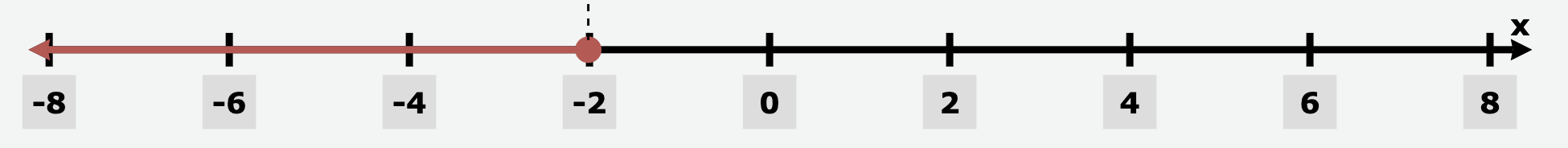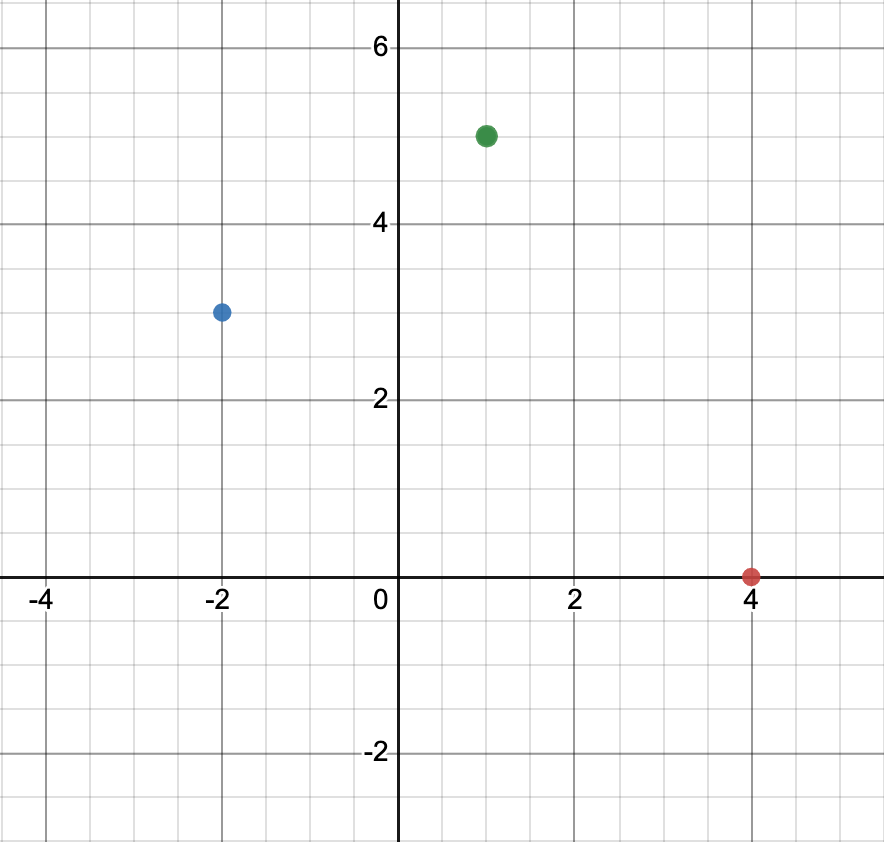Integer Operations
Equations
Graphing
Factors & Multiples
Potluck!
100

- 2 - 5 = ?

-7

100

x-13=27

x=40

100

Trivia Break!

I attend Grace from kindergarten through 12th grade. Can you guess when I graduated?

2013

100

List all the factors for the number 24

1, 24, 2, 12, 3, 8, 4, 6

100

4^3=?

64

200

Would the following product be positive or negative?

3*-4*-5*2*-11

Negative - there are three negative signs, so the product is also negative

200

y/3=-6

y=-18

200

Graph the inequality on a number line:

x ≤ -2200

Trivia Break!

What is my favorite animal?

a moose!

200

2^2+(-3+6)-11

-4

300

Trivia Break!

Until recently, I lived overseas in Europe. Where did I live?

Vienna, Austria

300

3x+5=-16

x=-7

300

Using an x/y table, create a graph for y=2x+1300

What is the greatest common factor for 16 and 36?

4

300

What is the square root of 144?

12

400

-3+5-10=?

-8

400

x/4-3=2

x=20

400

On a coordinate plane, I plotted the points (0,4), (-2, 3), and (1,5). Which, if any, were plotted incorrectly?(0, 4) was plotted incorrectly

400

What is the least common multiple of 4 and 12?

12

400

Trivia Break!

After high school, I went to college at a small Christian school in the lower 48. Can you guess which state? Bonus points if you can guess the college!

Michigan - Calvin University
500

-11*2+8=?

-14

500

Trivia Break!

Though I teach math at Grace (& yearbook), I have a degree in another subject as well. Can you guess which subject?

History

500

Using an x/y table, create a graph for y=3x500

What is the greatest common factor of 11 and 72?

1, because 11 is prime

500

You are shopping for new school clothes. A shirt you like at Old Navy is $24, but the sales associate says it is 40% off. How much will the shirt cost?$14.40

Click to zoom